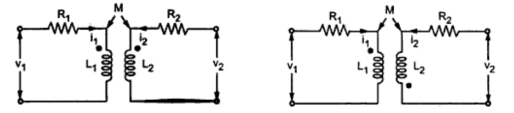### Dot Convention and Equilibrium Equations

The sign of mutually induced voltage depends on direction of winding of the coils. But it is very inconvenient to supply the information about winding direction of the coils. Hence dot conventions are used for purpose of indicating direction of winding. The dot conventions are interpreted as below :
1. If a positive current enters into the dots of both the coils or out of dots of both the coils, then mutually induced voltages for both the coils add to the self induced voltages hence mutually induced voltages will have same polarity as that of self induced voltages.
2. If a positive current enter into (or out of ) the dot in one coil and in other coil current flows out of (or into) the dot, then the mutually induced voltages will have polarity opposite to that of self induced voltages.
The dot conventions can be interpreted in simple words as follows :
1. If a current enters a dot in one coil, then mutually induced voltage in other coil is positive at the dotted end.
2. If a current leaves a dot in one coil, then mutually induced voltage in other coil is negative at the dotted end.
Consider the circuits shown in Fig. 1(a) and (b).Fig. 1 (a) and (b)

From Fig 1(a), v1 and v2 are voltages induced across coil L1 and L2 respectively and v1 and are v2 given by following equations,
v1 = iR1 + L1 (di1/dt) + M(di2/dt)
v2 = i2 R2 + L2 (di2/dt) + M(di1/dt)
From Fig. 1(b), current in coil 1 is entering the dot and current in coil 2 leaves the dot. Hence the voltages induced across coils L1 and L2 i.e. v1 and v2 are given by following equations,
v1 = iR1 + L1 (di1/dt) - M(di2/dt)
v2 = i2 R2 + L2 (di2/dt) - M(di1/dt)
1.1 Sign Convention for KVL
While applying Kirchhoff's voltage law the following sign convention is followed in this book :
1) When current flows through a circuit element it produces a voltage drop (+ -) across that element, in the direction of current as shown in the Fig.2. Thus across L1 there two voltages due to i1 and i2. Both produce drops in the respective directions.Fig. 2

2) When KVL is applied, the loop is to be traced in particular direction, either clockwise or anticlockwise.
3) While tracing a loop, if the polarities of voltage across an element is found to be + to - i.e. it is a drop then corresponding term must be taken negative in the equation.
4) While tracing a loop, if the polarities of voltage across an element is found to be - to + i.e. it appears as a potential rise then corresponding term must be taken positive in the equation.
5) All equations must be written in Σ V = 0 form.
Thus for circuit shown in the Fig. 2, the KVL for the loop ABEFA gives,
- i1 R1 - L1(di1/dt) + L1(di2/dt) + V = 0
While tracing in this direction, drops due to i1 appears as drops while drop due to i2 appears as a rise. This depends on the direction in which loop is traced. If same loop is traced as AFEBA then we get,
-V + L1(di1/dt) - L1(di2/dt) + i1 R1 = 0
But ultimately equation remains same.
Thus equation for loop BCDEB is,
-  i2 R2 -  L2(di2/dt) - L1(di2/dt) + L1(di1/dt)= 0
Note : In such equations, if M is given then the signs of L(di/dt) and M(di/dt) and M(di/dt) must be decided from the dot convention rules.
1.2 Writing Equilibrium Equations for Coupled Coils
Consider the circuit shown in the Fig. 3.Fig. 3

Now due to current i, the polarities of voltage drop across L1, L2 and R are as shown in the Fig. 3.
While terms related to M must be written considering dots.
Tracing loop a-c-d-b-a we get negative sign for the terms L1(di/dt), L2(di/dt) and iR.
As per dot convention, current leaving from the dotted end of one coil produces the negative at the dotted end of the other coil. Hence the terms of M(di/dt) must have signs opposite to self induced e.m.fs.
Hence KVL equation for loop a-c-d-b-a becomes
- L1(di/dt) + M(di/dt) - L2(di/dt) + M(di/dt) - iR + V = 0

Example 1 : Calculate effective inductance of the circuit shown (Fig. 4) across terminals a and b.Fig. 4

Solution : Assume that current ''i'' is flowing in series circuit and voltage developed across terminals a and b is shown in the following Fig. 4(a).Fig. 4(a)..

Applying KVL for the above circuit. The circuit flowing through all the coils is same i.e. ''i''.
While writing the equations follow the convention that the current entering in the dot of one coil produces positive at the dotted end of the the another coil while the current leaving from the dotted end of one coil produces the negative at the dotted end of the another coil.
- 4(di/dt) + 2(di/dt) - 5(di/dt) + 2(di/dt) - 2.5(di/dt) - 3(di/dt) - 2.5(di/dt) + v = 0
v = 13(di/dt)  = Leff (di/dt)
Effective inductance across terminals a and b is Leff.
Leff = 13 H